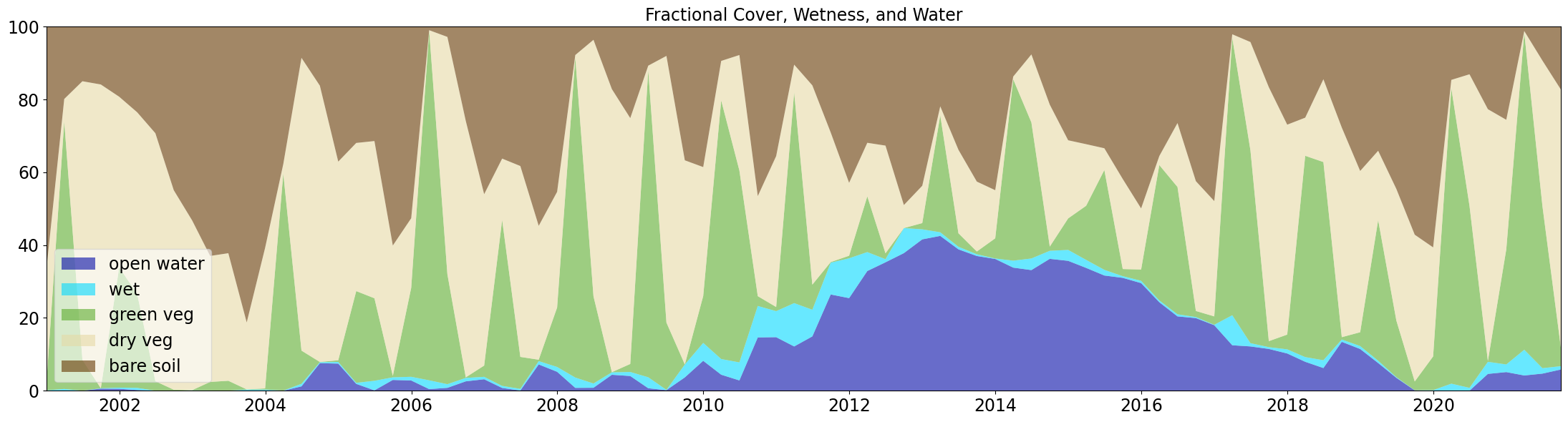# Monitoring wetlands in the Okavango¶

## Background¶

The Wetlands Insight Tool (WIT) provides insights into a wetland’s seasonal and interannual dynamics. The WIT is a spatiotemporal summary of an wetland that combines multiple datasets derived from the Landsat archive held within DE Africa. Fractional cover, WOfS, and Landsat surface reflectance data are retrieved from DE Africa’s ODC and combined to produce a stack plot describing the percentage of a wetland polygon as vegetation fractional cover, open water, and wet vegetation through time.

Detailed Explanation: The code calculates the Tasselled-Cap Wetness (TCW, or just ‘wetness’) from surface reflectance and takes the maximum Fractional cover fraction per pixel, masking Fractional cover with TCW, and masking TCW with open water. For each pixel inside or overlapping the polygon describing the wetland, WIT calculates the dominant fractional cover type. WIT selects the largest percentage value for each pixel as the dominant fractional cover type. Fractional cover was masked using WOfS and TCW to remove areas of water and wet vegetation from areas where fractional cover is calculated. This is necessary as the fractional cover algorithm erroneously classifies water as green vegetation (PV). The resulting output is a stacked plot of open water, wet vegetation, photosynthetic vegetation, non-photosynthetic vegetation, and bare soil for the wetland polygon through time.

## Description¶

This notebook will run the Wetlands Insight Tool for the area encompassed by a polygon

2. Run the Wetlands Insight Tool

3. Plot the results as a stacked line plot

### Getting started¶

To run this analysis, run all the cells in the notebook, starting with the “Load packages” cell. ***

:

# Force GeoPandas to use Shapely instead of PyGEOS
# In a future release, GeoPandas will switch to using Shapely by default.
import os
os.environ['USE_PYGEOS'] = '0'

import datacube
import pandas as pd
import seaborn as sns
import geopandas as gpd
import matplotlib.pyplot as plt
from datacube.utils import geometry

from deafrica_tools.wetlands import WIT_drill

from deafrica_tools.plotting import map_shapefile

[ ]:

create_local_dask_cluster()


### Analysis parameters¶

The following cell sets important parameters for the analysis:

• shp_path: a location path to the vector file (e.g. folder/input.shp).

• time_range: The date range to analyse (e.g. ('2015', '2019'))

:

shp_path = 'data/lake_ngami.geojson'

time_range = ('2000-12' , '2021-08')


We will also ensure the polygon is in WGS84 coordinates (epsg=4326) using the to_crs() method to make sure it can index the datacube correctly.

:

gdf = gpd.read_file(shp_path).to_crs('epsg:4326')


## Plot the polygon¶

This cell will plot the polygon over a basemap using the deafrica_tools.plotting function map_shapefile

:

#plot the shapefile over a basemap
gdf['id']=0
map_shapefile(gdf, attribute='id', fillOpacity=0.1)


## Run the Wetlands Insight Tool¶

Even for small areas, this code can take a long time to run so you will need to be patient. This is because the tool loads three seperate datasets (Landsat SR, Fractional Cover, and WOfS), and calculates a tasselled cap index on the fly.

If the region is large and/or the time-span is long, the cell below will take a long time to run. Use the Dask Dashboard to monitor the progress.

:

export_name='results/lake_ngami_WIT.csv'

:

df = WIT_drill(
gdf=gdf,
time=time_range,
min_gooddata=0.0,
TCW_threshold=-0.035,
resample_frequency="Q-DEC",
export_csv=export_name,
verbose=False,
)


/usr/local/lib/python3.10/dist-packages/rasterio/warp.py:344: NotGeoreferencedWarning: Dataset has no geotransform, gcps, or rpcs. The identity matrix will be returned.
_reproject(

:

wofs_area_percent wet_percent green_veg_percent dry_veg_percent bare_soil_percent
2000-12-31 0.00 0.11 1.14 31.66 67.09
2001-03-31 0.00 0.48 73.46 6.21 19.85
2001-06-30 0.00 0.00 8.48 76.54 14.98
2001-09-30 0.57 0.11 0.00 83.45 15.87
2001-12-31 0.53 0.31 33.02 46.90 19.23

### Plot the results¶

If you would like to export the plot as a .png file, then set the variable below to True, and give the plot a name (e.g. the wetland’s name)

:

export_plot = True
out_filename = 'results/Lake_Ngami_WIT.png'

:

fontsize = 17

# generate plot

#set up color palette
pal = [sns.xkcd_rgb["cobalt blue"],
sns.xkcd_rgb["neon blue"],
sns.xkcd_rgb["grass"],
sns.xkcd_rgb["beige"],
sns.xkcd_rgb["brown"]]

#make a stacked area plot
plt.clf()
fig=plt.figure(figsize = (22,6))
plt.stackplot(df.index,
df.wofs_area_percent,
df.wet_percent,
df.green_veg_percent,
df.dry_veg_percent,
df.bare_soil_percent,
labels=['open water',
'wet',
'green veg',
'dry veg',
'bare soil',
], colors=pal, alpha = 0.6)

#set axis limits to the min and max
plt.axis(xmin = df.index, xmax = df.index[-1], ymin = 0, ymax = 100)
plt.tick_params(labelsize=fontsize)
#add a legend and a tight plot box
plt.legend(loc='lower left', framealpha=0.6, fontsize=fontsize)
plt.title('Fractional Cover, Wetness, and Water', fontsize=fontsize)
plt.tight_layout();
if export_plot:
#save the figure
plt.savefig(out_filename);

<Figure size 640x480 with 0 Axes>Contact: If you need assistance, please post a question on the Open Data Cube Slack channel or on the GIS Stack Exchange using the open-data-cube tag (you can view previously asked questions here). If you would like to report an issue with this notebook, you can file one on Github.

Compatible datacube version:

:

print(datacube.__version__)

1.8.15


Last Tested:

:

from datetime import datetime
datetime.today().strftime('%Y-%m-%d')

:

'2023-08-11'